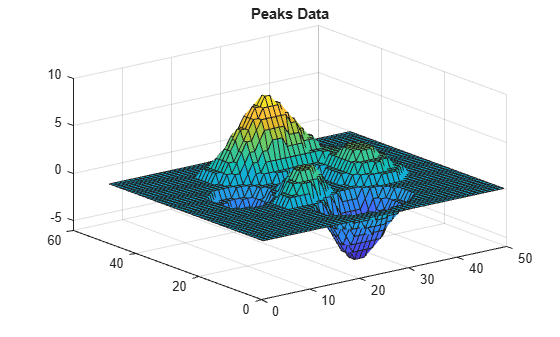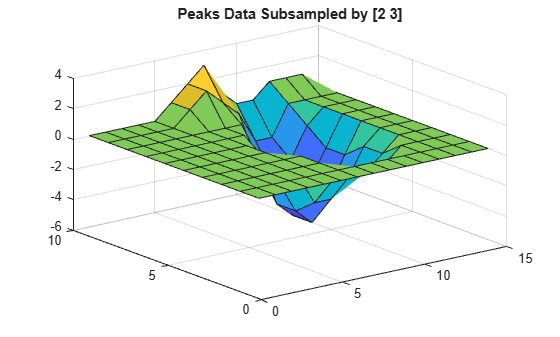Read data from variable in NetCDF data source

## Syntax

``vardata = ncread(source,varname)``
``vardata = ncread(source,varname,start,count)``
``vardata = ncread(source,varname,start,count,stride)``

## Description

example

``vardata = ncread(source,varname)` reads all the data from the variable `varname` contained in `source`.`

example

``vardata = ncread(source,varname,start,count)` reads data beginning at the location specified in `start`. The `count` argument specifies the number of elements to read along each dimension.`

example

``vardata = ncread(source,varname,start,count,stride)` returns data with the interval between the indices of each dimension of the variable specified by `stride`.`

## Examples

collapse all

Read and plot variable named `peaks` from the file `example.nc`.

```peaksData = ncread('example.nc','peaks'); whos peaksData```
``` Name Size Bytes Class Attributes peaksData 50x50 5000 int16 ```

Plot `peaksData` and add a title.

```surf(double(peaksData)); title('Peaks Data');```Read and plot only a subset of the variable data starting from the location `[25 17] `until the end of each dimension.

```startLoc = [25 17]; % Start location along each coordinate count = [Inf Inf]; % Read until the end of each dimension peaksData = ncread('example.nc','peaks',startLoc,count); whos peaksData```
``` Name Size Bytes Class Attributes peaksData 26x34 1768 int16 ```

Plot the data.

```surf(double(peaksData)); title('Peaks Data Starting at [25 17]');```Read and plot data, where the data is sampled at a specified spacing between variable indices along each dimension. Start reading from the location in `startLoc` and read variable data at intervals specified in `stride`. A value of `1` in `stride` accesses consecutive values in the corresponding dimension; a value of `2` accesses every other value in the corresponding dimension, and so forth.

```startLoc = [1 1]; count = [10 15]; stride = [2 3]; sampledPeaksData = ncread('example.nc','peaks',startLoc,count,stride); whos sampledPeaksData```
``` Name Size Bytes Class Attributes sampledPeaksData 10x15 300 int16 ```

Plot the data.

```surf(double(sampledPeaksData)); title('Peaks Data Subsampled by [2 3]');```## Input Arguments

collapse all

Name of the NetCDF data source, specified as a string scalar or character vector. The `source` argument can be one of these values:

• The path of a local NetCDF source

• The OPeNDAP URL of a remote OPeNDAP NetCDF data source

• The HTTP URL of a remote NetCDF source, with `#mode=bytes` appended to the end of the URL to enable byte-range reading

Note

Byte-range reading is slower than reading from other sources. For more details about byte-range reading, see the NetCDF documentation.

Example: `"myNetCDFfile.nc"`

Variable name, specified as a string scalar or character vector containing the name of a variable in the NetCDF data source.

Data Types: `string` | `char`

Starting location, specified as a numeric vector of positive integers. For an N-dimensional variable, `start` is a vector of length N containing 1-based indices.

If you do not specify `start`, then the `ncread` function starts reading the variable from the first index along each dimension.

Data Types: `double`

Number of elements to read, specified as a numeric vector of positive integers. For an N-dimensional variable, `count` is a vector of length N, specifying the number of elements to read along each dimension. If any element of `count` is `Inf`, then `ncread` reads until the end of the corresponding dimension.

If you do not specify `count`, then the `ncread` function reads the variable data until end of each dimension.

Data Types: `double`

Space between the variable indices along each dimension, specified as a numeric vector of integers. For an N-dimensional variable, `stride` is vector of length N. The elements of the `stride` vector correspond, in order, to the variable's dimensions. A value of `1` accesses adjacent values of the NetCDF variable in the corresponding dimension. Whereas, a value of `2` accesses every other value of the NetCDF variable in the corresponding dimension, and so on.

If you do not specify `stride`, then the `ncread` function reads the data with a default spacing of `1` along each dimension.

Data Types: `double`

## Output Arguments

collapse all

Variable data, returned as the data type that best matches the NetCDF data type of `varname`. For more information about how MATLAB® determines the best match, see More About.

For numeric data types, when at least one of the variable attributes `_FillValue`, `scale_factor`, or `add_offset` is present, then `ncread` returns `vardata` of type `double`. In addition, `ncread` applies these conventions:

• If the `_FillValue` attribute exists, then `ncread` replaces `vardata` values equal to `_FillValue` values with `NaN`s. If the`_FillValue` attribute does not exist, then `ncread` queries the NetCDF library for the fill value of the variable.

• If the `scale_factor` attribute exists, then `ncread` multiplies variable data by the value of the `scale_factor` attribute.

• If the `add_offset` attribute exists, then `ncread` adds the value of the `add_offset` attribute to the variable data.

Note

For variables of type `NC_CHAR`, the `ncread` function supports reading `vardata` that contains only ASCII-encoded characters. Reading `UTF-8` encoded characters is supported from variables of type `NC_STRING` in `NetCDF-4` files.

Data Types: `single` | `double` | `int8` | `int16` | `int32` | `int64` | `uint8` | `uint16` | `uint32` | `uint64` | `cell` | `char` | `string`

collapse all

### NetCDF to MATLAB Data Type Conversion

The NetCDF-related MATLAB functions automatically choose the MATLAB data type that best matches the NetCDF data type according to this table.

NetCDF Data TypeMATLAB Data Type
`NC_DOUBLE``double`
`NC_FLOAT``single`
`NC_INT64` (NetCDF-4 files only)`int64`
`NC_UINT64` (NetCDF-4 files only)`uint64`
`NC_INT``int32`
`NC_UINT` (NetCDF-4 files only)`uint32`
`NC_SHORT``int16`
`NC_USHORT` (NetCDF-4 files only)`uint16`
`NC_BYTE``int8`
`NC_UBYTE` (NetCDF-4 files only)`uint8`
`NC_CHAR``char`
`NC_STRING` (NetCDF-4 files only)`string`
User-defined `NC_VLEN` types (NetCDF-4 files only)`cell`

## Version History

Introduced in R2011a

expand all